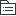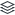# 资料分析

121
23

121
|
23

## 相关模板推荐

•## 资料分析 —— 作品大纲

•统计术语
• 增长相关
• 基期与现期
• 基期
• 识别：问题时间在材料之前
• 公式：基期=现期-增量=现期/（1+r）
• 现期
• 识别：问题时间在材料之后
• 公式：现期=基期+增量=基期*（1+r）
• 增长率与增长量
• 增长量
• 识别：增长+具体单位（元、吨、人）；最多或最少
• 公式：现期-基期=现期*r/（1+r）
• 增长率
• 识别：增长+%，倍，成；最快或最慢；增长速度，增长幅度
• 公式：增长率=增长量/基期量=（现期-基期）/基期=增长量/（现期-增长量）
• 易错点辨析
• 同比与环比
• 同比：与上年同期相比
• 环比：与紧紧相邻的上一时期相比
• 百分数与百分点
• 百分数表示两个量的比例关系
• 百分点表示两个百分数的差值
• 增长率、降幅、变化幅度
• 增长率：可正可负，且负的小于正的
• 降幅：必须要下降，再比较幅度
• 直接比较增长率的绝对值
• 增长了、增长到、翻番
• 增长（了）r倍=是原来的r+1倍
• 增长到n倍=就是原来的n倍
• 翻n番=2的n次方倍
• 比例相关
• 比重
• 比重指部分在总体中的比例关系
• 识别：题干中出现占、比重、贡献率、利润率、产销率
• 公式：“占”字一出现，前面除以后面；利润率=利润/收入，产销率=销量/产量
• 小贴士：资料分析中，利润=利润/收入；数学运算中，利润率=利润/成本
• 倍数
• 识别：是几倍，为几倍
• 公式：A是B的几倍，即A/B
• 提示：倍数就是增长率+1
• 平均
• 识别：平均、每、单位
• 公式：平均数=后面/前面 例：人均GDP=GDP/人数；每人消费=消费/人数；单位面积产量=产量/面积
• 其它术语
• 顺差、逆差
• 顺差=出口>进口；逆差=出口<进口
• 成数
• 几成就是十分之几 与折扣类似
• 翻番
• 翻1翻是2倍，翻N番=2的N次方倍
• 三大产业
• 第一产业：农业（种植业、林业、牧业、副业、渔业）
• 第二产业：工业和建筑业
• 第三产业：服务业
• GDP/GNP
• GDP：国内生产总值
• GNP：国民生产总值
• 恩格尔系数、基尼系数都是越小越好
•速算技巧
• 计算类（截位直除）
• 选项首位不同或首位相同次位差>首位： 除数四舍五入保留前两位有效数字
• 首位一样或次位差<=首位： 一般四舍五入保留前三位有效数字
• 比较类（分数比较）
• 分子大同时分母小，分数值大； 分子小且分母大的，分数值小。
• 分子分母同大同小时，看变化速度，变化快的起决定作用： 分子变化快则看分子，分子大则分数大； 分母变化快的看分母，分母大则分数值小。
•高频考点
• 增长类
• 增长量
• 计算
• 公式：增长量=现期-基期=基期*r=现期*r/（1+r）
• 计算技巧：r=1/n; 增长量=现期/（1+n）;减少量=现期/（n-1）
• 比较
• 当已知现期量和增长率：两者都大，增量肯定大
• 1+r差不多时，可直接比较现期*r
• 增长率
• 一般增长率
• 已知或问题为百分点 直接加减计算
• 公式：r=增量/基期量=（现期-基期）/基期=增量/基期
• 间隔增长率
• 中间隔一年的增长率，如：2015年比2013年增长百分之几
• 公式：r间隔r1+r2+r1*r2，其中当r1和r2均小于10%时可以忽略r1*r2。
• 混合增长率
• 混合增长率居中，但不正中，偏向基数较大的一方
• 线段法：基期两个部分的量之比与增长率之差成反比
• 年均增长率
• 计算：（r+1）的n次方=末期/初期 其中n表示年份差，计算一般结合代入法）
• 比较：年份差相同，直接比较：末期/初期
• 基期与现期
• 基期问题
• 公式：基期=现期/（1+r）; 间隔基期=现期/（1+间隔r）
• r大：截位直除法；r小化除为乘法。 基期和差：计算利用排除法
• 现期问题
• 增量不变：现期=基期+增量*n
• r不变：现期=基期*（1+r）的n次方
• 比例类
• 倍数
• A是B的多少倍
• 现期倍数
• 基期倍数【a和b分别代表A和B的增长率】
• 比重
• 部分占整体
• 现期倍数
• 基期比重【a和b分别代表A和B的增长率】
• 两期比重
• 升降：若a>b,比重上升；若a<b,比重下降；a=b，比重不变
• 具体计算结合排除：两期比重差一定小于部分和总体增长率差的绝对值
• 平均数
• 平均/每/单位
• 现期平均
• 基期平均（a和b分别代表A和B的增长率）
• 两期平均比较
• 当分子增长率>分母增长率，现期平均数大； 当分子增长率<分母增长率，现期平均数小。
• 两期平均数增长量
• 平均数的增长率
• 其他
• 简单计算
• 找到数据直接选或简单加减
• 排序题
• 注意顺序，看清是低到高还是高到低；
• 名词的坑，是增长率还是增长量等
• 单位，看清单位是万还是亿
• 大数据显示一般不选A
• 综合分析
• 简单入手，从现期、题干短的、计算简单的入手
• 大数据显示一般CDB正确概率依次递减，A选项的正确概率相对较低